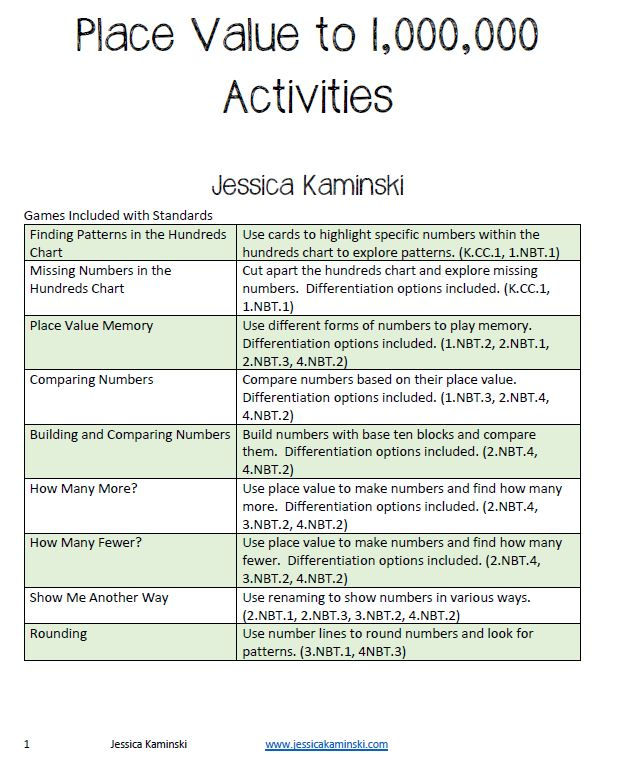Search
•Jessica Kaminski

# Place Value is Where It All Starts

Almost every textbook I've seen includes place value in the first few chapters. This is purposely placed at the beginning to root our students in the patterns and organization of our number system. Students will use their understanding of place value as they add, subtract, multiply or divide.

Starting at the end of Grade 1, students begin to understand the concepts of our base ten system. They begin to explore the concepts of renaming 10 of one place for 1 of another. These seemingly simple tasks help shape efficiency in operations.### Digging Deeper into Place Value

Are your students grasping these big ideas from their place value learning?

• A digit in each place value has a specific value based on its location.

• Only digits 0-9 can be placed in the place value chart EXCEPT when regrouping.

• Numbers can be renamed for other numbers. 245 can be 2 hundreds, 4 tens, 5 ones or even 1 hundred, 14 tens and 5 ones and so many other ways. Choosing which version we need can help us with mental math.

• Patterns exist in the place value chart. When multiplying by 10, 100, or 1000, numbers move to the left on the place value chart. When dividing by 10, 100, or 1000 numbers move to the right. Knowing these patterns can help us check whether the answer is reasonable.

• Rounding is so much more than a bunch of rules. If I use counting skills to explore number lines, I can easily see the rounded number.

Learning about place value can be so much fun while tackling an essential topic. Encourage students to play hands-on games that can even be adapted for single players for social distancing by grabbing this free download by clicking below: